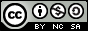- 目录 -
LeetCode 1111 有效括号的嵌套深度(找规律)

输入：seq = "(()())"



输入：seq = "()(())()"



1 <= text.size <= 10000


• 空字符串
• 连接，可以记作 AB（A 与 B 连接），其中 A 和 B 都是有效括号字符串
• 嵌套，可以记作 (A)，其中 A 是有效括号字符串

• s 为空时，depth("") = 0
• s 为 A 与 B 连接时，depth(A + B) = max(depth(A), depth(B))，其中 A 和 B 都是有效括号字符串
• s 为嵌套情况，depth("(" + A + “)”) = 1 + depth(A)，其中 A 是有效括号字符串

## 代码

  1 2 3 4 5 6 7 8 9 10 11 12 13 14 15 16 17  class Solution { public: vector maxDepthAfterSplit(string seq) { vector res; int depth = 0; for (int i = 0; i < seq.size(); i++) { if (seq[i] == '(') res.push_back((++depth) & 1); else res.push_back((depth--) & 1); } return res; } };